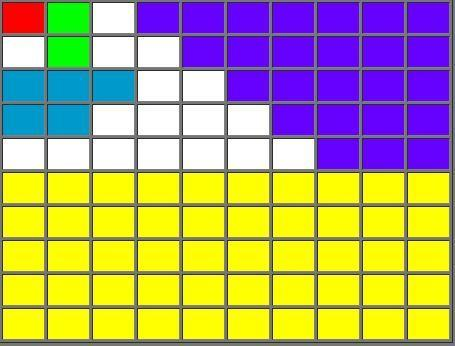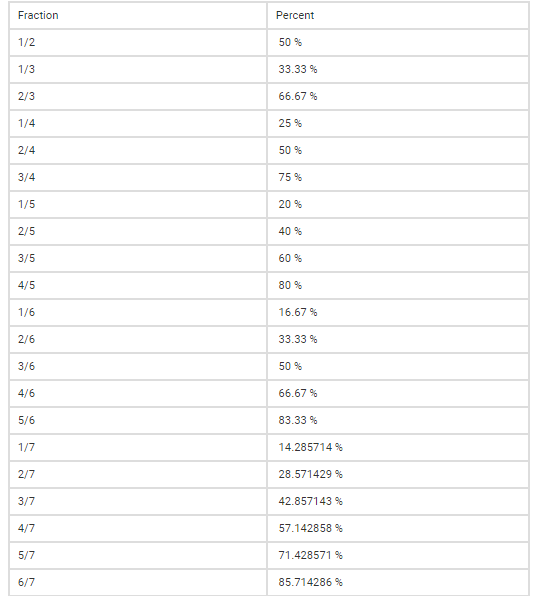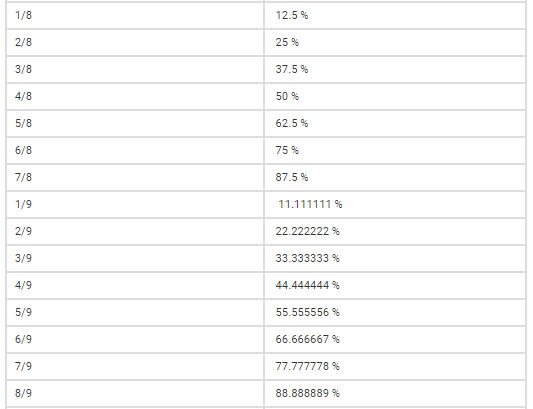Top

# Percentage

CONCEPT 1:

Understanding Percentages.

• Percent comes from the Latin word “per centum”. The Latin word “centum” means 100, so “per centum” means “for each hundred”. Percent is denoted with a symbol “%”. For instance, 1 percent can be written as 1%, and it is the same as the fraction 1/100, Ten percent can be written as 10%, which is the same as the fraction 10/100.

• When we say percent, we mean a part of the whole where whole is considered as hundred. Look at the grid below with 100 cells to understand better.

• Each cell is equal to 1% of the whole (the red cell is 1%).

• Two cells are equal to 2% (the green cells).

• Five cells are equal to 5% (the blue cells).

• Twenty-five cells (purple cells) are equal to 25% of the whole or one quarter (¼).

• Fifty cells (yellow cells) are equal to 50% of the whole or half (½).• Now, Can you calculate how many unshaded (white) cells are there? What is the percentage of unshaded cells? There are three ways to work this out. Look at the following ways:

• Count the white cells. There are 17 of them. Out of 100 cells, 17% are therefore white.

• Add up the number of other cells and deduct them from 100. There is one red cell, two green, five blue, twenty-five purple, and fifty yellow. That adds up to 83. So, (100−83) = 17. Again, out of 100 cells, 17 are white, or 17%.

• Divide the value of white cells by total value and then multiply the resultant to 100. Percentage= (Value of white cells/Total value) ×100 OR 17/100 × 100 = 0.17 × 100 = 17 or 17%.

• Percentage is textually written as, Percentage= (Part/Whole) × 100. Now, this part & whole can be any value, but when we convert this ratio in percentage, we mean to define the resultant out of every hundred. This gives us freedom to express complex calculations in simple form. For example, If I say 20% of my shirt was torn, a person listening to this will get an idea about portion of shirt that was torn without even knowing actual length of the shirt and length of the portion that was torn because when I said 20% of my shirt was torn, he considered the whole shirt as hundred cells and imagined that 20 cells out of it were torn. Therefore, we can easily communicate in terms of percentage without expressing detailed calculations.

CONCEPT 2:

Conversion of fraction into percentages and vice versa.

• To convert a fraction into percentage, just multiply the given ratio by 100, the result obtained will be in terms of percentage. Example: To express 2/3 in terms of percentage, we multiply the given ratio by 100 or (2/3) × 100 = 66.66%.

• Similarly, if we want to convert a number given in terms of percentage, we divide the number by 100. Example: To express 40% in terms of ratio, we divide 40 by 100 or (40/100) = 2/5.

• Now, look at the percentage-fraction conversion chart below. This will help you solve problems quickly.CONCEPT 3:

Percentage increase or decrease.

• Percentage increase is the ratio of value increased to the original value and multiplied by 100. It is expressed in percentage. If there is an increase in the value of anything, then there is an increase in percentage.

• Value Increased   × 100 = Percentage Increase.

Original Value

• Let us understand this with an example. If your salary is Rs.50,000/- per month and one day your company’s HR walks in and informs you that you are promoted to senior management and your new salary will be Rs.60,000/-. What is the percentage increase or hike on your salary?

• Solution:  We can clearly see that there is an increase in your salary which is Rs.10,000/-. So, what will be the percentage increase? Percentage increase as defined above can be written as (10000/50000) * 100 which is equal to 20%. So, you received a 20% hike on your current salary.

• Percentage decrease is the ratio of value decreased to the original value and multiplied by 100. It is expressed in percentage. If there is a decrease in the value of anything, then there is a decrease in percentage.

• Value Decreased × 100 = Percentage Decrease.

Original Value

• Let us understand this with an example. Last month your total expenditure was Rs.20,000/-. However, this month you have managed your expenses well and your total expenditure was Rs.15,000/-. What is the percentage decrease on your expenditure?

• Solution: We can clearly see that there is a decrease in your expenditure as compared to previous month which is Rs.5000/-. So, what percentage will be percentage decrease? Percentage decrease as defined above can be written as (5000/20000) * 100 which is equal to 25%. So, you reduced your expenses by 25%.

• An important point to note while calculating percentage increase or decrease is that the value increased or decreased is always calculated on original value and not on resultant value. This is a common mistake people do.

CONCEPT 4:

Understanding the concept of multiplying factor for quick calculations.

• Let’s learn a unique approach to ease the calculation while solving percentage increase or decrease. This will also help you in solving general problems related to percentages very quickly.

• What do we mean when we say that a value has increased by 20%? As discussed earlier, we consider total value of anything or it’s whole part as hundred or 100%. Now, if we say that this whole value is increased by 20%, we mean that a value which was 100% earlier has now increased by 20% or it has become 120% of the original value. 120% can be further written as 120/100 or 1.2. This “1.2” works out to be your multiplying factor. Multiplying 1.2 to your original value will give you the increased value.

• What do we mean when we say that a value has decreased by 20%? We mean that a value which was 100% earlier has now decreased by 20% or it has become 80% of the original value. 80% can be further written as 80/100 or 0.8. This “0.8” is your multiplying factor. Multiplying 0.8 to your original value will give you the decreased value.

• Using concept of multiplying factor will help you save time from lengthy calculations through formula. Let’s us understand this through few examples.

• If the current salary of an employee working in ABC company is Rs.24000/-. What will be his new salary if he received a hike of 20% on his salary?

• Solution: According to given question, the employee gets hike of 20% on his salary. So, according to formula:

Value Increased × 100 = Percentage Increase

Original Value

Value increased = (20 × 24000)/ 100 or Rs.4800/- or you could simply calculate 20% of 24000= 4800 (without using formula).

New salary of the employee will be 24000+4800 = Rs.28,800/-

• Can we make this simpler? Yes! By using concept of multiplying factor. Here you go.

 24000 × 1.2 = 28,800

Did we just get the answer in single line? How did we do that? Simple! Salary of the employee was Rs.24000/- which can be considered has 100% of his salary as this amount represents the whole part of his salary. Now according to question, his salary increased by 20% which means now his salary is 120% of the original salary or we can express it as:

(120% of 24000) or 24000 × (120/100) or 24000 × 1.2.

• Let’s quickly solve few problems.

• Increase 5000 by 23%.

• Increase 200 by 200%.

• Increase 17400 by 12%.

• Decrease 2800 by 13%

• Decrease 5000 by 20%.

• Decrease 12000 by 15%.

• Express ¾ in terms of percentage.

• Express 7/8 in terms of percentage.

• Express 15/14 in terms of percentage (round off the value)

• Express 125% in terms of fraction.

• Express 160% in terms of fraction.

• Express 285% in terms of fraction.

CONCEPT 5:

Few tricks to calculate faster.

• Splitting the percentage into parts.

• Example: Find 51% of 128.

• Solution: 51% of 128= (50+1) % of 128= 50% of 128 + 1% of 128 = 64 + 1.28 = 65.28

• Interchanging the percentage value and the number.

• Example: Find 39% of 12.5.

• Solution: 39% of 12.5 = 12.5% of 39 = 1/8 × 39 = 4.875

PROBLEMS ON PERCENTAGE

FOUNDATION

1. What is 15 % of Rs. 34?

1. Rs. 3.40

2. Rs. 3.75

3. Rs. 4.50

4. Rs. 5.10

5. None of these

2. What percent of Rs. 2650 is Rs. 1987.50?

1. 60 %

2. 75 %

3. 80 %

4. 85 %

5. None of these

3. If 35 % of a number is 12 less than 50 % of that number, then the number is:

1. 40

2. 50

3. 60

4. 80

5. None of these

4. A person’s salary has increased from Rs. 7200 to Rs. 8100. What is the percentage increase in his salary?

1. 25 %

2. 18 %

3. 16 2/3 %

4. 12 1/2 %

5. None of these

5. 15 % of 10 % of 20 % of 1000 is?

1. 1.50

2. 67

3. 150

4. 3

5. None of these

MODERATE

1. In an examination it is required to get 35 % of the aggregate marks to pass Rishu got 216 marks and declared failed by 5 % marks then what was the total marks?

1. 620

2. 720

3. 820

4. 710

5. None of these

1. The price of Petrol went up 20 %. In order to keep expenses same Ram must reduce travel by:

1. 25 %

2. 30 %

3. 33.33 %

4. 16.67 %

5. None of these

1. If the length and breadth of a rectangle field are increased. The area increased by 50 %. If the length increased by 20 %, by what percentage was breadth increased?

1. 25 %

2. 30 %

3. 20 %

5. None of these

1. Aditya’s salary is 80 % of Amit’s salary and 120 % of Rajiv’s salary. What is Amit’s salary if Rajiv’s salary is 30,000?

1. 40000

2. 45000

3. 50000

4. 55000

5. None of these

1. In a class 60 % of the students pass in Hindi and 45 % pass in Sanskrit. If 25 % of them pass in both subjects, what percentage of the students fails in both subjects?

1. 80 %

2. 20 %

3. 25 %

4. 75 %

5. None of these

HOTS -HIGH ORDER THINKING SKILLS

1. Ram borrowed Rs. 725 from Shyam at the beginning of a year at interest. After 8 months, he again borrowed Rs. 362.50 at a rate of interest double that the former sum bears. At the end of the year, the sum of interest on both loans is Rs. 43.50. Find the first rate of interest per annum?

1. 4.5 %

2. 4.75 %

3. 6.25 %

4. 7.2 %

5. None of these

2. The cost price of goods with a bankrupt is Rs. 25500 and if the goods had realised in their full value, his creditors would have received 85 paise in the rupee. But 2/5 of the goods were sold at 17 % and the remainder at 22 % below their cost price. How many paise in a rupee was received by the creditors?

1. 72 paise

2. 68 paise

3. 55 paise

4. 52 paise

5. None of these

3. A shopkeeper undertakes to supply 2000 tables at Rs. 1725 each. He estimates that if 10 % are defective which will be sold at 50 %, then the profit will be 15 % on his whole outlay. When the tables were supplied, 70 % of the tables were found defective. What loss did the shopkeeper incur?

1. Rs. 607500

2. Rs. 557500

3. Rs. 550500

4. Rs. 80680

5. None of these

4. Sweta invested Rs. 10000 in a scheme exactly three years ago. The value of the investment increased by 10 % during the first year, increased by 5 % during the second year, and decreased by 10 % during the third year. What is the value of the investment today?

1. Rs. 10500

2. Rs. 10395

3. Rs. 10342

4. Rs. 10230

5. None of these

5. In Mumbai, 60 % of the registered voters are BJP supporters and the rest are Congress supporters. In a mayoral race, if 75 % of the registered voters who are BJP supporters and 20 % of the registered voters who are congress supporters are expected to vote for candidate X, what percent of the registered voters are expected to vote for candidate X?

1. 53 %

2. 55 %

3. 57 %

4. 59 %

5. None of these

SOLUTIONS

FOUNDATION

1. (d) (15 / 100) × 34 = Rs. 5.10

2. (b) Required percentage = (252 / 270) × 100 = 93 1/3 %

3. (d) Let the number is X (50 / 100) X – (35 / 100) X = 12 » 15X / 100 = 12 » X = (12 × 100) / 15 » X = 80

1. (d) Percentage increase in salary = (900 / 7200) × 100             = 100/8 = 12 ½ %

2. (d) (15 / 100) × (10 / 100) × (20 /100) × 1000 = 3

MODERATE

1. (b) If Rishu failed by 5 % marks which means he got 30 % marks. 30 % marks = 216 » 100% marks = (216/30) × 100 Total marks = 720

2. (d) If the price of petrol has increased by 20 %, it has gone up 1/5th of its earliest price. .’. The % of reduction in petrol that will maintain the amount of money spent on petrol = 1/ (1+5) = 1/6 = 16.67% » Reduction in travel = 16.67%

3. (a) Let the increased breadth be X » 20 + X + 20X/100 = 50 [% increase = m+n+mn/100] » X + X/5 = 30 » 6X/5 = 30 » X = (30 × 5)/6 » X = 25%

4. (b) Let Amit’s salary be X Aditya salary = (120/100) × 30000 = Rs. 36000 (X × 80)/ 100 = 36000 » X = (36000 × 100)/80 =Rs. 45000

5. (b) The percentage of students fails in both subjects = 100 – [(60 + 45) – 25] = 20 %

HOTS- HIGH ORDER THINKING SKILLS

1. (a) 43.5 = (725 × R × 1)/100 + (362.5 × 4 × 2R)/ (12 × 100) 43.5 × 300 = 2175R + 362.5 × 2R = 2900 R R = 4.5

2. (b) Total debt = 22500 × 100/85 = Rs. 30000 Money received by selling the goods = 25500 [(2/5) × (83/100) +(3/5) × (78/100)] = 25500/500 × (166 + 234) = 51 × 400 = Rs. 20400 Therefore, money received by the creditors for a rupee = Rs. 20400/30000 = Rs. 0.68 = 68 paise Hence, the creditor received 68 paise in a rupee

3. (a) 10% 0f 2000 = 200 Selling price of 200 tables at 50% =Rs. 200 × 1725/ 2 = Rs. 172500 Selling price of remaining 1800 tablet = Rs. 1800 × 1725 = Rs. 3105000 Total revenue from selling 2000 tables = Rs. 172500 + 3105000 = Rs. 3277500 Now, Rs. 3277500 includes 15 % profit. Therefore, cost price of 2000 tables = (100/115) × 3277500 = Rs. 2850000 Now the actual selling price = 2000 × (30/100) × 1725 + 2000 × (70/100) × (1725/2) = 2000 × 1725 [(30/100) + (35/100)] = 20 × 1725 × 65 = Rs. 2242500 .’. Loss = Cost price – Selling price Hence, the shopkeeper incurs a loss of » Rs. 2850000 – Rs. 2242500 = Rs. 607500

4. (b) The first year’s increase of 10% can be expressed as 1.10; the second year’s increase of 5% can be expressed as 1.05; and the third year’s decrease of 10% can be expressed as 0.90. Now, multiply the original value of the investment account by each of these yearly changes. 10000 × 1.10 × 1.05 × 0.90 =Rs. 10395 Hence, the value of the investment today is = Rs. 10395.

(a) Let Y be the number of registered voters in Mumbai. Then, the information that 60% of the registered voters are from BJP can be expressed as 0.60Y. From this, it can be stated that 1.00Y – 0.60Y = 0.40Y are from Congress. The percentage of BJP supporters and the percentage of Congress supporters who are expected to vote for candidate X can be expressed as: X = 0.75 × 0.60Y + 0.20 × 0.40Y = 0.45Y + 0.08Y = 0.53Y Hence, 53% of the registered voters are expected to vote for candidate X.